Thermodynamics and Propulsion

# 8.1 Behavior of Two-Phase Systems

The definition of a phase, as given by SB&VW, is a quantity of matter that is homogeneous throughout.'' Common examples of systems that contain more than one phase are a liquid and its vapor and a glass of ice water. A system which has three phases is a container with ice, water, and water vapor.

We wish to find the relations between phases and the relations that describe the change of phase (from solid to liquid, or from liquid to vapor) of a pure substance, including the work done and the heat transfer. To start we consider a system consisting of a liquid and its vapor in equilibrium, which are enclosed in a container under a moveable piston, as shown in Figure 8.1. The system is maintained at constant temperature through contact with a heat reservoir at temperature, so there can be heat transfer to or from the system.

 [][][]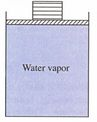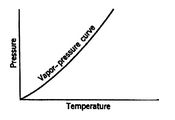For a pure substance, as shown in Figure 8.2, there is a one-to-one correspondence between the temperature at which vaporization occurs and the pressure. These values are called the saturation pressure and saturation temperature (see Ch. 3 in SB&VW).

This means there is an additional constraint for a liquid-vapor mixture, in addition to the equation of state. The consequence is that we only need to specify one variable to determine the state of the system. For example, if we specifythen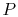is set. In summary, for two phases in equilibrium,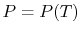. If both phases are present, any quasi-static process at constantis also at constant.

Let us examine the pressure-volume behavior of a liquid-vapor system at constant temperature. For a single-phase ideal gas we know that the curve would be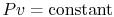. For the two-phase system the curve looks quite different, as indicated in Figure 8.3.Several features of the figure should be noted. First, there is a region in which liquid and vapor can coexist, bounded by the liquid saturation curve on the left and the vapor saturation curve on the right. This is roughly dome-shaped and is thus often referred to as the vapor dome.'' Outside of this regime, the equilibrium state will be a single phase. The regions of the diagram in which the system will be in the liquid and vapor phases respectively are indicated. Second is the steepness of the isotherms in the liquid phase, due to the small compressibility of most liquids. Third, the behavior of isotherms at temperatures below the critical point'' (see below) in the region to the right of the vapor dome approach those of an ideal gas as the pressure decreases, and the ideal gas relation is a good approximation in this region.

The behavior shown is found for all the isotherms that go through the vapor dome. At a high enough temperature, specifically at a temperature corresponding to the pressure at the peak of the vapor dome, there is no transition from liquid to vapor and the fluid goes continuously from a liquid-like behavior to a gas-type behavior. This behavior is unfamiliar, mainly because the temperatures and pressures are not ones that we typically experience; for water the critical temperature isand the associated critical pressure is 220 atmospheres.

There is a distinct nomenclature used for systems with more than one phase. In this, the terms vapor'' and gas'' seem to be used interchangeably. In the zone where both liquid and vapor exist, there are two bounding situations. When the last trace of vapor condenses, the state becomes saturated liquid. When the last trace of liquid evaporates the state becomes saturated vapor (or dry vapor). If we put heat into a saturated vapor it is referred to as superheated vapor. Nitrogen at room temperature and pressure (at one atmosphere the vaporization temperature of nitrogen is 77 K) is a superheated vapor.Figure 8.4 shows lines of constant pressure in temperature-volume coordinates. Inside the vapor dome the constant pressure lines are also lines of constant temperature. It is useful to describe the situations encountered as we decrease the pressure or equivalently increase the specific volume, starting from a high pressure, low specific volume state (the upper left-hand side of the isotherm in Figure 8.3). The behavior in this region is liquid-like with very little compressibility. As the pressure is decreased, the volume changes little until the boundary of the vapor dome is reached. Once this occurs, however, the pressure is fixed because the temperature is constant. As the piston is withdrawn, the specific volume increases through more liquid evaporating and more vapor being produced. During this process, since the expansion is isothermal (we specified that it was), heat is transferred to the system. The specific volume will increase at constant pressure until the right hand boundary of the vapor dome is reached. At this point, all the liquid will have been transformed into vapor and the system again behaves as a single-phase fluid. For water at temperatures near room temperature, the behavior would be essentially that of a perfect gas in this region. To the right of the vapor dome, as mentioned above, the behavior is qualitatively like that of a perfect gas.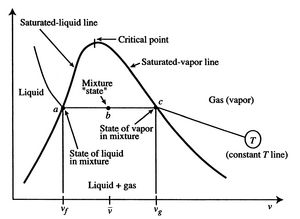Referring to Figure 8.5, we define notation to be used in what follows. The statesand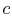denote the conditions at which all the fluid is in the liquid state and the gaseous state respectively.

The specific volumes corresponding to these states are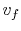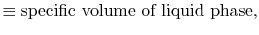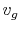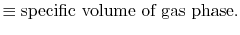For conditions corresponding to specific volumes between these two values, i.e., for state, the system would exist with part of the mass in a liquid state and part of the mass in a gaseous (vapor) state. The average specific volume for this condition isWe can relate the average specific volume to the specific volumes for liquid and vapor and the mass that exists in the two phases as follows. The total mass of the system is given byThe volume of the system is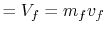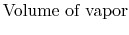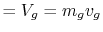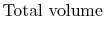The average specific volume,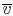, is the ratio of the total volume to the total mass of the system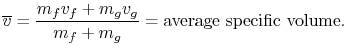The fraction of the total mass in the vapor phase is called quality, and denoted by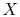: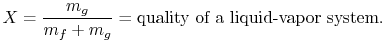In terms of the quality and specific volumes, the average specific volume can be expressed as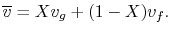In reference to Figure 8.6,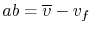,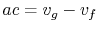.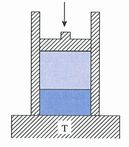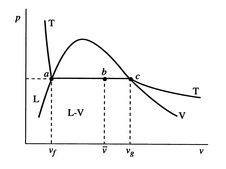UnifiedTP